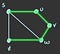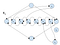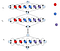# Hamiltonian Path is NP-Complete

Presented By Georgia Gans and Lucia Vaccaro

Original proof by Adrian She can be found at http://www.cs.toronto.edu/~ashe/ham-path-notes.pdf

# Introduction

If we’re going to talk about the Hamiltonian Path problem, we should probably talk about graph theory first. In mathematics, graph theory is the study of, well, graphs. But when we talk about graph theory, we aren’t talking about what may first come to mind when we think of graphs (i.e., functions, the unit circle, calculus, etc.). In graph theory, a graph is a structure made up of a set of vertices (also referred to as nodes) and a set of edges which connect pairs of those vertices. Further, a directed graph is one where there is a direction associated with each edge, so we may only traverse from one vertex to another if the direction of the edge between them will allow it.

In computer science, graph theory has some really important applications. Graphs are often utilized as a data structure and they provide great insight into the connectivity of anything and everything. They are a great tool for answering many questions, like: How can a GPS find the shortest route to your destination? Who should Facebook recommend to be your friend? What is the longest path around the country where you are able to visit every major city? And, most importantly, does this graph have a Hamiltonian Path?

# What is a Hamiltonian Path?

Now that we know a little bit about graph theory, we can talk about what it means for a graph to have a Hamiltonian Path. A graph G has a Hamiltonian Path from some vertex s to another vertex t if there is a path that connects the two vertices which touches each vertex in the graph exactly once.The picture above shows an example of a Hamiltonian Path. The highlighted green edges exhibit the path from the vertex s to the vertex t.

# The Problem

The problem that we will be discussing today is often referred to as HAMPATH, and it is the problem of determining if a directed graph has a Hamiltonian Path from a vertex s to another vertex t. More specifically, we will be overviewing the proof that this problem is NP-complete. That is, HAMPATH is in the NP class and it is NP-hard.

# The Proof

As with any NP-completeness proof, this one has two parts. First, we will go over why HAMPATH is in the NP class. To be in NP means that, given a proposed solution (a certificate) to the problem, we can verify the solution in polynomial time. In this case, that means that given a directed graph G and path between 2 vertices, we can check in polynomial time if the path is a Hamiltonian Path.

If we are given a certificate, we can do the following to algorithmically check for a Hamiltonian Path:

The above algorithm takes polynomial time, and so HAMPATH is in NP.

For the second part of the proof, we will go over why HAMPATH is NP-hard. For a language to be NP-hard means that any language in NP is polynomial time reducible to it. So, to show that HAMPATH fits this description, we will show the reduction from a known NP-complete language 3SAT. Recall that

3SAT = {φ | φ is a satisfiable Boolean formula in 3CNF}

So, in order to complete this reduction, we want to take some element φ of 3SAT and translate it into a graph G such that φ is satisfiable if and only if G has a Hamiltonian Path. So, first, let φ be of the form (a₁៴ b₁ ៴ c₁) ∧ (a₂ ៴ b₂ ៴ c₂)∧ . . . ∧(ak ៴ bk ៴ ck) where each (aᵢ, bᵢ ,cᵢ) is a clause xᵢ and there are k total clauses. What we want to do from here is build a “gadget”, which is essentially a small graph, for each variable (a₁, b₁, etc.) and for each clause and then connect them together such that they satisfy our goal.

So, let’s first create a variable gadget. We can start by drawing one vertex. Each variable can be either true or false, so we want to have two other vertices connected to the original one, each representing a path we can take depending on the variable’s value. But, if we want this small graph to have a Hamiltonian Path, we need those two new nodes to be connected as well. To solve this problem, we are going to add 2k vertices between the two outer vertices in the middle row to make a total of 2k+2 nodes in the row.

These vertices will connect the two we drew earlier and have two directed paths, one going each way. If the variable is designated as true, then we will traverse the path from left to right and if the variable is designated as false then we will traverse from right to left. Finally, those two “outer” vertices we drew are going to have one more edge coming off of each of them, connecting at a singular vertex below. The purpose of this is to make it easier to chain together our gadgets later.

One of these gadgets will be made for every variable in the boolean formula φ. Then, each gadget is chained together vertically, so the bottom vertex of one becomes the top vertex of the next.

In addition to this set of gadgets strung together, there is also an additional set of k C-nodes (clause-nodes) that exist outside of the gadgets. Each C-node represents a clause in 3SAT. If a variable xᵢ is mentioned in a clause, there will be a path drawn to the corresponding C-node from left to right. If the complement of xᵢ is mentioned in the clause, there will be a path drawn from right to left. These constitute what we earlier referred to as a clause gadget.

Each row of nodes must have a way to link to each C-node so we can keep track of which variables are connected to which clauses. Since it takes two nodes to make a path, each variable uses two nodes to represent each clause.This image is a gadget of x₁, where x₁ is a variable in an arbitrary 3CNF statement. X₁ is in clause 1 and the complement of x₁ is in clause 3. X₁ is not present in clause 2.

Lastly, let the vertex at the very top of the graph be labeled s, and let the vertex at the very bottom be t. This concludes the construction of the graph G.The figure above shows what the global structure of the graph G looks like. It shows some elements of G and their relationships, except the edges that represent the relationship of the variables to the clauses that contain them.

Now that we have constructed the graph, we want to show that it is indeed the case that φ is satisfiable if and only if there is a Hamiltonian Path in G from s to t.

First, assume that φ is satisfiable, and we wish to show that this means there is a Hamiltonian Path from s to t. Then, we can visit each vertex, starting at s. In each variable gadget, we traverse left to right if the variable is true, and right to left if it is false. This is now where the C-nodes come in. If φ is satisfiable, which we assumed it is, then at least one variable in each clause must be true and we will therefore reach the corresponding C-node. Therefore, all vertices will be visited because we will traverse all the ones in each variable gadget as well as each C-node.

Then, assume that G has a Hamiltonian Path from s to t, and we wish to show that this means that φ is satisfiable. If G has this Hamiltonian Path, then by definition the path touches every vertex, including the C-nodes. By the construction of the graph, then, we see that at least one variable in each clause must be true in order for the traversal to include each C-node. When at least one variable in each clause is true, all of φ is true and therefore φ is satisfiable.

Hence, with this construction of a graph G, φ is satisfiable if and only if there is a Hamiltonian Path in G from s to t. Further, this reduction happens in O(kn) where k is the number of clauses and n is the number of variables. This is polynomial time and so HAMPATH is indeed NP-hard. Therefore, HAMPATH is NP-complete.

# Discussion

Overall this proof is very useful, but it was difficult to understand at first. By studying this proof, we are better able to understand the Hamiltonian Path as a concept and its relationship to other graph theory problems. It showed us that you can turn virtually anything into a graph, which emphasized how versatile graphs and graph theory as a whole can be, and now it makes more sense when people say that graphs are everywhere in computer science.

This proof shows us that it is possible to make a “machine” that tells us if there’s a Hamiltonian Path on a graph in polynomial time. Being able to tell if there exists a path between any two given vertices that passes through every other vertex on the graph exactly once can be very useful in fields that use graphs in any context. This problem has applications in computer graphics, electric circuitry, and other various domains.

One thing to note about the graph that is constructed through this proof is that it is very complex, which can be both a good and a bad thing. Because the graph has so many edges, it is very clear that there is a path between each vertex in the main structure, and therefore easier to see that whether or not the graph has a Hamiltonian Path solely depends on the C-nodes. However, because of the sheer number of edges it is hard to tell what exactly is going on in each gadget.

One shortcoming of this proof is that it is very inefficient. This graph has many, many nodes and many, many edges and passing this graph through any type of algorithm on a computer seems like it would be very tedious.

Since this proof/problem is very well-known and considered foundational in computational theory, we don’t believe that the proof itself has any shortcomings. That being said, we do think that many representations of the proof are very complicated and difficult to understand for someone who is new to the topic, and we believe it could be simplified in future iterations.

# Group Contributions

Georgia found the article. We both wrote the slideshow and did the video together. Georgia drafted the first part of the blog post (intro and first part of proof explanation) and Lucia drafted the second part of the proof explanation and the discussion, and also made the graphics in the blog post. We both edited the blog post over together.